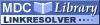Search
Browse
Statistics
Feeds

# Fundamental Study - Enumerating all connected maximal common subgraphs in two graphsItem Type: Article Fundamental Study - Enumerating all connected maximal common subgraphs in two graphs Koch, I. We represent a new method for finding all connected maximal common subgraphs in two graphs which is based on the transformation of the problem into the clique problem. We have developed new algorithms for enumerating all cliques that represent connected maximal common subgraphs. These algorithms are based on variants of the Bron-Kerbosch algorithm. In this paper we explain the transformation of the maximal common subgraph problem into the clique problem. We give a short summary of the variants of the Bron-Kerbosch algorithm in order to explain the modification of that algorithm such that the detected cliques represent connected maximal common subgraphs. After introducing and proving several variants of the modified algorithm we discuss the runtimes for all variants by means of random graphs. The results show the drastical reduction of the runtimes for the new algorithms. Bron-Kerbosch Algorithm, Cliques, Connected Maximal Common Subgraphs, Graph Theory Theoretical Computer Science 0304-3975 Elsevier 250 1-2 1-30 6 January 2001 https://doi.org/10.1016/S0304-3975(00)00286-3 View item in PubMed

Repository Staff Only: item control page

Open Access
MDC Library